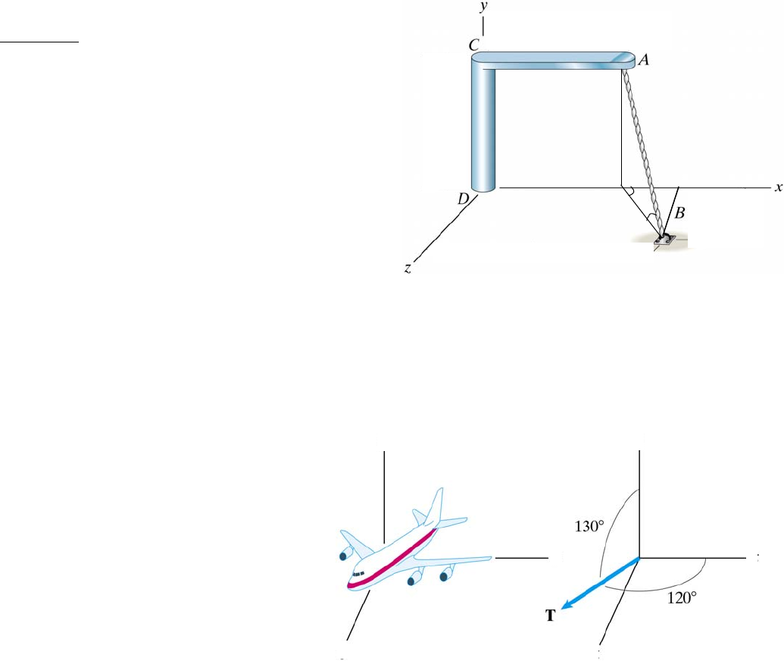# ENGG202 Lecture 1: Tutorial 3 Solutions.pdf

29 views4 pagesENGG 202 Tutorial 3 January 22/23, 2013
* question is from a past ENGG 205 exam
3D vectors
1*) The line CA is contained in the x-y plane. Point
B lies in the x-z plane. Point O is located directly
under point A. Express the unit vector directed along
the line AB in Cartesian vector format given that the
angle OBA (α) is 65o and angle xOB (β) is 40o.
ANS: uAB = 0.324i + -0.906j + 0.272k
Solution Strategy: First, recognize that we are asked
to find components for the vector directed from A to
B, not from B to A. Since we want components of a
unit vector then just take the magnitude of the vector
as 1. Using the angle α, and considering the right triangle AOB, determine 2 components, 1 that is
on line AO and is parallel to the y-axis (so it is the y component) and 1 that is parallel to line OB.
Now break the OB component into 2 further components using the angle β, and considering the
right triangle HOB. 1 component will be parallel to the x axis (x component) and 1 is parallel to
the z axis (z component).
2*) The airplane’s engines exert a total
thrust force T with a magnitude of 200 kN.
The angle between T and the y axis is 120o,
and the angle between T and the z axis is
130o. The x component of T is positive.
(a) What is the angle between T and the x
axis?
(b) Express T in Cartesian vector format.
ANS: θ = 54.5o
T = (116.1i + -100.0j + -128.6k) kN
Soln Strategy: the two angles shown are two of the coordinate angles (angle between T and the
y and z axes. The third angle can be found from the relationship: cos2θx + cos2θy + cos2θz =1.
(direction cosines are the components of a unit vector). Note that using this equation (which
involves squares) will not give you the correct sign of the direction cosine so you have to pay
attention to whether the x component is positive (angle should be less than 90) or negative (angle
should be between 90 and 180). With all the angles know the components are simply: Tx=
Tcosθx , Ty= Tcosθy , Tz= Tcosθz
α
β
O H
x
z
y
z
y
x
Unlock document

This preview shows page 1 of the document.
Unlock all 4 pages and 3 million more documents.